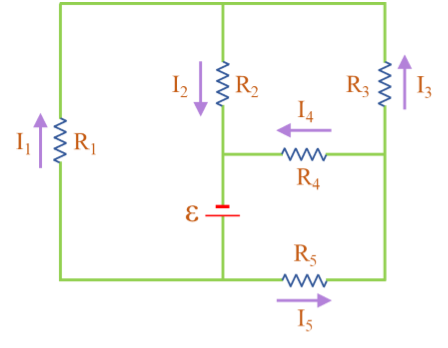# In the circuit shown below: \varepsilon = 64.0 V, R_5 = 4.00 Ohms, R_3 = 2.00 Ohms, R_2 = 2.20...

## Question:

In the circuit shown below:

{eq}{\LARGE\varepsilon} \, = \, 64.0 \, V, \quad R_5 \, = \, 4.00 \, \Omega, \quad R_3 \, = \, 2.00 \, \Omega, \quad R_2 \, = \, 2.20 \, \Omega, \quad I_5 \, = \, 9.20 \, A, \quad I_4 \, = \, 6.10 \, A, \quad \text{and} \quad I_1 \, = \, 12.70 \, A. {/eq}

Find the current through {eq}\, R_2 \, {/eq} and {eq}\, R_3 {/eq}, and the values of the resistors {eq}\, R_1 \, {/eq} and {eq}\, R_4 {/eq}.## Kirchhoff's Circuit Laws

According to Kirchhoff's voltage law, in a closed loop with resistors, the sum of voltage drop across the resistors should equal the battery emf i.e.

{eq}V \ = \ \Sigma \ I_i \times R_i {/eq}

According to Kirchhoff's current law, the sum of current going into a node should equal the sum of currents coming out of it.

{eq}\Sigma \ I_{in} \ = \ \Sigma \ I_{out} {/eq}

Become a Study.com member to unlock this answer! Create your account

Given:

• Resistors: {eq}R_2 \ = \ 2.2 \ \Omega {/eq}, {eq}R_3 \ = \ 2 \ \Omega {/eq} and {eq}R_5 \ = \ 4 \ \Omega {/eq}
• Current: {eq}I_1 \ = \ 12.7...Kirchhoff's Law: Definition & Application

from

Chapter 12 / Lesson 7
40K

Kirchhoff's laws can be used to analyze complex circuits. In this lesson, you will learn about Kirchhoff's two laws and how to apply them to circuit analysis.